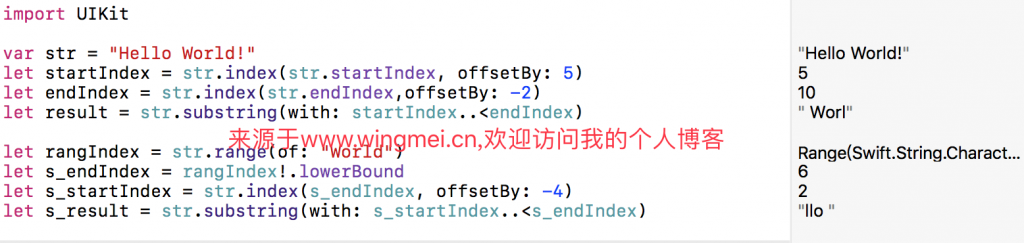## JavaFX,Unity3D,Android,IOS等技术教程和生活随笔，仅供记录

http://www.wingmei.cn/wp-content/themes/Vtrois-Kratos-e85a527/images/background.jpg

# IOS Swift 3.1字符串截取

``````func substring(from: String.Index)
func substring(to: String.Index)
func substring(with: Range)``````

``````var str = "Hello World!"
let startIndex = str.index(str.startIndex, offsetBy: 5)
let endIndex = str.index(str.endIndex,offsetBy: -2)
let result = str.substring(with: startIndex..<endIndex)``````

``````let rangIndex = str.range(of: "World")
let endIndex = rangIndex!.lowerBound
let startIndex = str.index(endIndex, offsetBy: -4)
let result = str.substring(with: startIndex..<endIndex)````````````+(NSString *)subString:(NSString *)content startIndex:(int)startIndex length:(int)length {
NSString* result = [content substringWithRange:NSMakeRange(startIndex, length)];
return result;
}``````